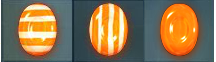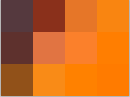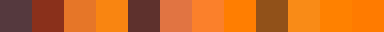Hello again, I am really lazy with posting stuff, but due to popular demand (2 friends) I decided to offer a more in-depth explanation regarding the candy crush bot that I made. I did start writing this post quite some time ago, but things always got in the way of me finishing it. Now remember that this is an explanation for beginners so if you do know the basics you can skip it and check out the code on github.

First of all you can check out the initial explanation here:

The part that wasn’t really well explained in the youtube movie was the one about the machine learning algorithm that was used to detect the candy type. I’ll focus on that here and I will try to show you the steps that I went through while trying to come up with a solution.

As I mentioned in the movie this bot performs the following tasks: 1) Grabbing a desktop screenshot 2) Cropping out the game board 3) Cropping out each cell of the game board 4) Detecting the candy types for each cell 5) Finding a move that would give us the highest reward 6) Sending input to the window and performing the move 7) Wait for the board image to stabilize. 8) Check for the end-game screen. If not found goto 1 :)

## Step 1-3

Nothing fancy going on in here. I just took a screenshot of the desktop and from it I extracted the regions of interest using some hard-coded the screen coordinates that I found using paint. We could do this in a smarter way, but I don’t really want it to be easy for people to use the bot in order to play the game :) . This was done mainly for teaching purposes and I believe that there are more important parts to this project than usability.

## Step 4 - Candy detection

Now we have the board cells and we need to know what candy is in each cell.As you can see we need to distinguish an orange candy from an orange candy with vertical stripes and an orange candy with horizontal stripes. This seems tricky bit it’s actually not. First let’s go back to the basics.

What is a picture? Let’s zoom in and consider that a picture is just a collection of colors in the shape of a matrix like this:Zooming in we see this:
rgb | rgb | rgb
rgb | rgb | rgb
rgb | rgb | rgb

We can flatten out this data structure putting each row one after the other and we get:What if we consider it like the array below? What does this look like?
r,g,b,r,g,b,r,g,b,r,g,b,r,g,b,r,g,b,r,g,b,r,g,b,r,g,b

What if we have less numbers in there? Ex: (r,g,b) Well this looks a lot like: (x, y, z). After all we have 3 numbers in there, names don’t matter.

So if that is a point in a three-dimensional space that means that (r,g,b,r,g,b,r,g,b,...) can be considered a point in an N-dimensional space. Well that’s a bit hard to imagine :) . Thankfully we don’t need to imagine this, we just need to use that insight in order to reach a solution.

How big is N for our picture? Well it’s Width*Height*3 (3 for r, g, b). But for the time being, let’s go back to 2-dimensional space.

What can we do if we have two points in space ? Let’s say we have the two points:

$$A = (x_1, y_1) B = (x_2, y_2)$$

We can get the distance between these two points using the well-known formula:

$$d = \sqrt{( x_1- x_2 )^2 + (y_1-y_2)^2}$$

In N dimensions this becomes:

$$A = (a_1, a_2, a_3, a_4, ... ,a_n) B = (b_1, b_2, b_3, b_4, ..., b_n) d(A, B) = \sqrt{(a_1-b_1)^2 + (a_2-b_2)^2 + (a_3-b_3)^2 + (a_4-b_4)^2 + ... (a_n-b_n)^2}$$

So if we introduce a third point C in the mix we can determine if C is closer to A or B using the formula above.

Notice how we did not say anything about pictures until now? Well that’s because my solution is simple and it doesn’t care that the data that we feed it is a picture. It just treats it as an array of numbers. Of course, if we know that we are dealing with pictures, we could do smarter stuff, such as feature detection or tons of other fun algorithms, but I’m not doing that, I’m just basically comparing distances.

Moving on, now that we established the simple rules of our world concerning distances we just need to gather some data that we can use for “training”. My first solution just loaded the pictures that are listed above and for each label it computed a center point (the mean for all the pictures - n dimensional points - in a folder). New pictures will be compared to that center and that is how I found out what type the new candy was.

What are the downsides of such an approach? First of all speed is not that great, remember one picture is quite big (71x63 pixels. This means we had a 13419 - dimensional point) After implementing the solution described above I decided that I needed something more reliable and faster.

Scikit-learn features a lot of great machine learning algorithms and we can pick from any of them and try them out and see what works best. I chose SVM.svc - support vector machines. You can read more about them here

This made it faster, but we should not stop here. Let’s think how we can make this even faster. One option would be to use downsized pictures when doing the training and prediction. Instead of using the original 71x63 crops, we can easily distinguish between candies even when using 32x32 pictures (a point in 3072 (32x32x3) dimensions). We could also go lower than that, but I settled for 32x32.

Now the most important methods for this are the following:

def train(self):
if os.path.isfile('svc.dat'):
#just load a previously saved classifier
else:
np_data = np.array(self.training_data)
np_values = np.array(self.target_values)
self.svc.fit(np_data, np_values)
#save it for later
joblib.dump(self.svc, 'svc.dat', compress=9)

def predict(self, img):
resized_img = img.resize(self.downscale_res, Image.BILINEAR)
np_img = np.array(resized_img.getdata()).flatten()
return int(self.svc.predict(np_img))


Skit-learn classifiers have two very important methods: fit and predict. Fit will train the classifier by using some example data that is labeled, and predict will give us the label using the information previously gathered with fit. This is called supervised machine learning, meaning that the training data was labeled. The other kind of learning is unsupervised learning where you have a bunch of unlabeled data and the classifier tries to sort it out into categories.

self.load() just loads the training data and prepares self.training_data (training points) and self.target_values (labels) arrays.

Profiling also showed that even with scikit learn calling fit took quite a lot of time. We know what the data is so there is no point in training the classifier each time we start playing a game since the training data did not change. This is what we cache in svc.dat in the code above.

You can check out the full code in here

## Step 5 - Finding a good move

Now that we have a game board represented in the form of a matrix where each cell is the candy type we can try and come up with an algorithm that will maximize the reward.

In the web game, new candies spawn randomly from the top of the table. So planning is out of the question.

How can we determine what the best move is? Well that is easy - try out all the moves, give each one a score, and pick the highest scoring one.

Can we come up with a better algorithm? I don’t think so. It has actually been shown that candy-crush is NP-hard. We could do better if we knew what candies drop from the top of the board when other candies break. In that case this turns in a search problem and we can plan a bit ahead. But for now let’s use this greedy solution where we just pick the best move for the current board. The board is small enough and trying out all possible moves is not a big performance impact.

Scoring each move is based on some really crude reverse-engineering of the game rules. I just played the game and observed the following: - More candies crushed means more points. - Chocolate candies break all candies of a certain color - Vertically striped candies break everything in a vertical line - Horizontally striped candies break a whole row - You can match any 2 special candies to obtain even more points and effects

I did not accurately simulate all of this, but the results seemed ok most of the time and so the current (not very precise) solution stuck.

Now let’s take a break and think about something. What if the board was impossibly big? Then some interesting questions arise: Should we start from the bottom of the board ? Would starting from the center give us better results ? Should we start from the same place each time ? Some interesting heuristics could be applied in this case and trying stuff out and analyzing the data would be a fun project.

## Step 6 - Sending input to the game.

This turned out to be quite easy. I couldn’t find an easy way that worked for multiple platforms, but on windows you can do it using the following methods:

def win32_click(x, y):
win32api.SetCursorPos((x, y))
win32api.mouse_event(win32con.MOUSEEVENTF_LEFTDOWN, x, y, 0, 0)
win32api.mouse_event(win32con.MOUSEEVENTF_LEFTUP, x, y, 0, 0)

def do_move(move):
start, end = move

start_w = get_desktop_coords(start)
end_w = get_desktop_coords(end)
#save the original cursor position
initial_pos = win32api.GetCursorPos()

win32api.SetCursorPos(start_w)
win32api.mouse_event(win32con.MOUSEEVENTF_LEFTDOWN, start_w, start_w, 0, 0)
time.sleep(0.3)
win32api.SetCursorPos(end_w)
time.sleep(0.3)
win32api.mouse_event(win32con.MOUSEEVENTF_LEFTUP, end_w, end_w, 0, 0)

#set the cursor back to the original position
win32api.SetCursorPos(initial_pos)


I am sure that there is a counterpart for other platforms that works in a similar way, but I did not investigate it. Sorry :).

## Conclusion

First of all congratulations in reaching the end of this quite lengthy post. I hope that the things presented above proved useful and might help you in investigating and coding some fun projects on your own. If you have any questions or comments feel free to leave them below.